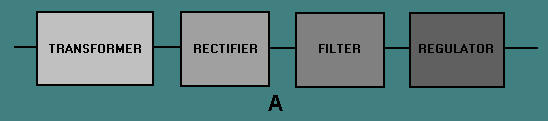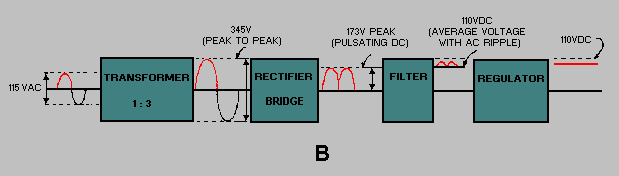Custom SearchSOLID-STATE POWER SUPPLIES LEARNING OBJECTIVES Upon completion of this chapter you will be able to: Identify the various sections of a power supply. State the purpose of each section of a power supply. Describe the operation of the power supply from both a whole unit standpoint and from a subunit standpoint. Describe the purpose of the various types of rectifier circuits used in power supplies. Describe the purpose of the various types of filter circuits used in power supplies. Describe the operation of the various voltage and current regulators in a power supply. Describe the operation of the various types of voltage multipliers. Trace the flow of ac and dc in a power supply, from the ac input to the dc output on a schematic diagram. Identify faulty components through visual checks. Identify problems within specific areas of a power supply by using a logical isolation method of troubleshooting. Apply safety precautions when working with electronic power supplies. In today's Navy all electronic equipment, both ashore and on board ship, requires a power supply. The discovery of the silicon diode and other solid-state components made possible the reduction in size and the increase in reliability of electronic equipment. This is especially important on board ship where space and accessibility to spare parts are a major concern. In this chapter, you will read about the individual sections of the power supply, their components, and the purpose of each within the power supply. THE BASIC POWER SUPPLY View A of figure 4-1 shows the block diagram of a basic power supply. Most power supplies are made up of four basic sections: a TRANSFORMER, a RECTIFIER, a FILTER, and a REGULATOR. Figure 4-1A. - Block diagram of a basic power supply.As illustrated in view B of figure 4-1, the first section is the TRANSFORMER. The transformer steps up or steps down the input line voltage and isolates the power supply from the power line. The RECTIFIER section converts the alternating current input signal to a pulsating direct current. However, as you proceed in this chapter you will learn that pulsating dc is not desirable. For this reason a FILTER section is used to convert pulsating dc to a purer, more desirable form of dc voltage. Figure 4-1B. - Block diagram of a basic power supply.The final section, the REGULATOR, does just what the name implies. It maintains the output of the power supply at a constant level in spite of large changes in load current or input line voltages. Now that you know what each section does, let's trace an ac signal through the power supply. At this point you need to see how this signal is altered within each section of the power supply. Later on in the chapter you will see how these changes take place. In view B of figure 4-1, an input signal of 115 volts ac is applied to the primary of the transformer. The transformer is a step-up transformer with a turns ratio of 1:3. You can calculate the output for this transformer by multiplying the input voltage by the ratio of turns in the primary to the ratio of turns in the secondary; therefore, 115 volts ac X 3 = 345 volts ac (peak-to-peak) at the output. Because each diode in the rectifier section conducts for 180 degrees of the 360-degree input, the output of the rectifier will be one-half, or approximately 173 volts of pulsating dc. The filter section, a network of resistors, capacitors, or inductors, controls the rise and fall time of the varying signal; consequently, the signal remains at a more constant dc level. You will see the filter process more clearly in the discussion of the actual filter circuits. The output of the filter is a signal of 110 volts dc, with ac ripple riding on the dc. The reason for the lower voltage (average voltage) will be explained later in this chapter. The regulator maintains its output at a constant 110-volt dc level, which is used by the electronic equipment (more commonly called the load). Q.1 What are the four basic sections of a power supply?Q.2 What is the purpose of the rectifier section?Q.3 What is the purpose of the filter section?Q.4 What is the purpose of the regulator section?